# Calculate the Bar Bending Schedule For Retaining Wall

## Calculate the Bar Bending Schedule For Retaining Wall

Today I will explain The Bar bending schedule for the retaining wall.bar bending schedule means the all detail of re bar use in foundation or in retaining wall. which is the diameter of bar length of bar weight of bar and location of bar is mentioned in BBS. Calculate the Bar Bending Schedule For Retaining Wall Bar Bending Schedule For Retaining Wall | BBS For Retaining Wall.

Now we calculate the bar bending schedule for the retaining wall.

### Given data

Length of Retaining wall = 9 m

With of wall = 0.300 m

Height of Wall = 2.500 m

Length of Foundation = 9 m

Width of foundation = 2.150 m

Height of Foundation= 0.400 m.

Concrete Cover = 50 mm

Spacing of Foundation Bar =150 mm

Spacing of wall vertical Bar = 150 mm

Spacing of wall distribution bar =150 mm

Spacing of Link Bar =300 mm

Dia of main bar of foundation = 16 mm

Dia of distribution bar of foundation =10 mm

Dia of vertical bar of wall inside = 16 mm

Dia of vertical bar of wall outside =12 mm

Dia of distribution bar of wall =10 mm

Dia of link bar of wall =8 mm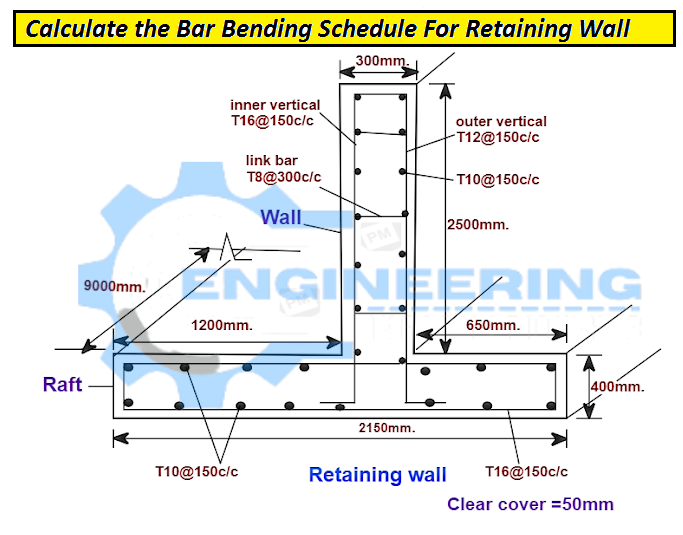### Cutting length of Foundation Main Bar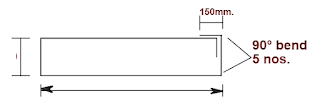Cutting length = 2 x(width + height ) – 5 x 90 degree bend  (90 degree bend = 2d = 2 × 16 =32 mm)

Width = foundation width – 2 concrete cover

Width = 2150 – 2×50 =2050 mm

Height = foundation height – 2 concrete cover

Height = 400- 2 x 50 =300 mm

Cutting length = 2 (2050+300) – 5×32 =4540=4.540 m

Total length of main bar = No’s of bar x cutting length of bar

Number of bar = total length of foundation /spacing +1

Number of bar = 9000/150 +1 =61 no’s

Total length of main bar =61 x 4.540 =276.94 m

### Weight of main Bar of foundation

Weight = d²/162.25 x length

Weight = (16)²/162.25 x 276.94 =433.80 kg=434 kg

### Cutting Length of Foundation Distribution Bar

Cutting length= length of retaining wall – 2 x concrete cover

Cutting length= 9000 – 2 x 50 =8900 mm =8.900 m

Total length of Foundation Distribution bar =No’s of bar x cutting length

Number of bar = total width /spacing +1

Number of bar = 2150/150+1= 15.33=16 no’s

Total length of Foundation Distribution bar = 16 x 8.900 =142.4 m

### Weight of Foundation Distribution bar

Weight = d²/162.25 x total length

Weight = (10)²/162.25 x 142.40 = 87.765 kg = 88 kg

### Vertical bar inside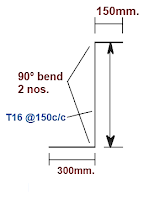### Cutting length of vertical bar inside

Cutting length =  T/B bend +total height of wall and foundation – T/B concrete cover – 2 x 90º bend( 90º bend 2×2d =2×16 =64 mm)

Cutting length vertical bar inside = (300+150) + ( 2500+400)- 2×50 -64 =3.186 m

Total length of vertical bar inside = No’s of bar x cutting length

Number of bar = total height of wall /spacing +1

Number of bar = 9000/150+1 =61 No’s

Total length of vertical bar inside =61 x 3.186 =194.346 m

### Weight of vertical bar inside

Weight =d²/162.25 x total length

Weight = (16)²/162.25 x 194.346=306.641 kg=365 kg

### Cutting length of vertical bar outside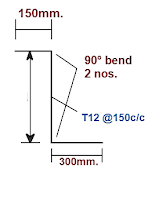Cutting length =  T/B bend +total height of wall and foundation – T/B concrete cover – 2 x 90 degree bend( 90 degree bend 2×2d =2×2×12 =48 mm)

Cutting length vertical bar outside = (300+150) + (2500+400) – 2×50 -48=3.202 m

Total length of vertical bar outside = No’s of bar x cutting length

Number of bar = total height of wall /spacing +1

Number of bar = 9000/150+1 =61 No’s

Total length of vertical bar outside =61 x 3.202 =195.322 m

### Weight of vertical bar inside

Weight =d²/162.25 x total length

Weight = (12)²/162.25 x 195.322=173.35=174 kg

### Cutting length of Distribution Bar of wall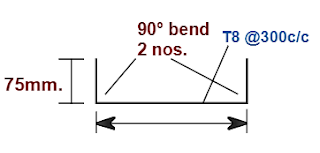Cutting length of distribution bar of wall=length of retaining wall – 2 concrete cover

Cutting length of distribution bar of wall= 9000 – 2×50 =8900=8.900 m

Total length of Distribution Bar of wall= No’s of bar x cutting length

Number of Distribution Bar of wall= height /spacing +1

Number of Distribution Bar of wall= 2500/150+1=18 No’s

Total length of Distribution Bar of wall= 18×8.900 =160.2 m

### Weight of Distribution Bar of wall

Weight of Distribution Bar of wall = d²/162.25 x total length

Weight = (10)²/162.25 x 160.2 =98.73=99 kg

### Cutting length of Link Bar of wall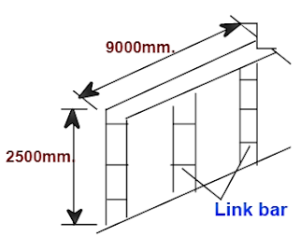Cutting length =  width of wall+2 bends -2 x concrete cover -2 x 90 degree bends (2 x 90 degree bends =2 x 2d=2×2×8=32)

Cutting length =  300+2×75 -2×50 – 32=318 mm=0.318 m

Total length = No’s of bar x cutting length

Number of bar along length side = total length /spacing +1

Number of bar =9000/300+1=31 Nos

Number of bar along height side = 2500/300 +1=9.33=10 no’s

Total number of bar = 31+10=41 nos

Total length = 41 x 0.318=13.038 m

Weight = d²/162.25 x total length

Weight =(8)²/162.25 x 13.038=5.142 kg=16 kg

### BBS Of The Retaining Wall

 S.No. Type of Bar Dia in mm. No’s Length in m. Total length in m. Weight in Kg/m. Total bar wt.in kg. 1. Main bar of foundation 16 61 4.54 276.94 1.578 434 2. Distribution bar of foundation 10 16 8.90 142.4 0.617 88 3. Vertical bar of wall 4 Inside vertical bar of wall 16 61 3.186 194.346 1.578 307 5 Outside vertical bar of wall 12 61 3.202 195.322 0.888 174 6 Distribution bar of  the wall 10 18 8.90 160. 0.617 99 7 Link bar of the wall 8 41 0.318 13.038 0.395 6 Total weight of the bar 1017 Add 3% of the wastage 30.51 The  total wt. of re bar’s 1047.51

### Raja Numan

Hi, My name is Engr. Raja Numan author of Engineering Information Hub and I am a Civil Engineer by Profession and I've specialized in the field of Quantity Surveying, Land Surveying as QC Engineer in national and multinational companies of Pakistan & Saudi Arabia.

### One Comment

1.Harry Forchick says:

I found you website very educative. I see a lot of important information

error: Content is protected !!